# 807 and Level 1

What can I say about the number 807?

807 is palindrome 151 in BASE 26 because 1(26²) + 5(26) + 1(1) = 807.

Anything else? Well, I can figure out a few other things because 807’s has two prime factors, 3 and 269:

We can write ANY number (unless it’s a power of 2) as the sum of consecutive numbers in at least one way. 807 has three different ways to do that:

• 403 + 404 = 807 because 807 isn’t divisible by 2.
• 268 + 269 + 270 = 807 because it is divisible by 3.
• 132 + 133 + 134 + 135 + 136 + 137 = 807 since it is divisible by 3 but not by 6.

I know that one of 807’s factors, 269, is a hypotenuse of a Pythagorean triple, so 807 is also. Thus. . .

• (3·69)² + (3·260)² = (3·269)², or in other words, 207² + 780² = 807²

Since 807 has two odd sets of factor pairs, I know that 807 can be written as the difference of two squares two different ways:

• 136² – 133² = 807
• 404² – 403² = 807

I don’t usually do this, but today’s puzzle has something in common with 807. Can you tell what it is?Print the puzzles or type the solution on this excel file: 10-factors 807-814

• 807 is a composite number.
• Prime factorization: 807 = 3 x 269
• The exponents in the prime factorization are 1 and 1. Adding one to each and multiplying we get (1 + 1)(1 + 1) = 2 x 2 = 4. Therefore 807 has exactly 4 factors.
• Factors of 807: 1, 3, 269, 807
• Factor pairs: 807 = 1 x 807 or 3 x 269
• 807 has no square factors that allow its square root to be simplified. √807 ≈ 28.4077454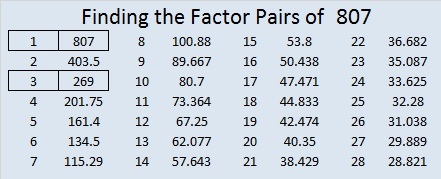# 804 Is There a Pot of Gold at the End of This Rainbow?

Factor Rainbows can be a wonderful way to display the factors of a number. Not only are all the factors listed in order from smallest to greatest, but the factor pairs are joined together with the same color band.

The number 804 has 12 factors so it makes a lovely rainbow with 6 different color bands.Is there a pot of gold at the end of this factor rainbow? I’ll let you decide the answer to that question.Print the puzzles or type the solution on this excel file: 10-factors 801-806

Finding golden nuggets of information about a number might be less difficult than finding pots of gold.

I always begin the painstaking mining process by looking at the factors of the number:

• 804 is a composite number.
• Prime factorization: 804 = 2 x 2 x 3 x 67, which can be written 804 = (2^2) x 3 x 67
• The exponents in the prime factorization are 2, 1, and 1. Adding one to each and multiplying we get (2 + 1)(1 + 1)(1 + 1) = 3 x 2 x 2 = 12. Therefore 804 has exactly 12 factors.
• Factors of 804: 1, 2, 3, 4, 6, 12, 67, 134, 201, 268, 402, 804
• Factor pairs: 804 = 1 x 804, 2 x 402, 3 x 268, 4 x 201, 6 x 134, or 12 x 67
• Taking the factor pair with the largest square number factor, we get √804 = (√4)(√201) = 2√201 ≈ 28.3548937575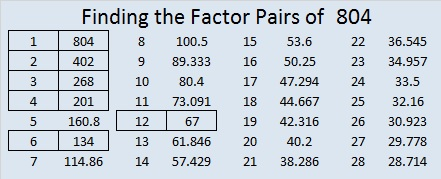Finding nuggets of information about the number 804 has been a little difficult and disappointing:

• None of 804’s prime factors can be written as 4N+1, so 804 is NOT the hypotenuse of any Pythagorean triples.
• 804 is NOT a palindrome in base 36 or any base less than that.
• 804 is NOT the sum of any consecutive prime numbers.

Even though I did not find any golden nuggets in those places, I kept looking and finally found a couple of gems about the number 804:

804 can be written as the sum of three squares four different ways, and all of those ways have some definition of double in them:

• 28² + 4² + 2² = 804
• 26² + 8² + 8² = 804
• 22² + 16² + 8² = 804
• 20² + 20² + 2² = 804

OEIS.org also gives us a nugget about the number 804 that may be a bit too heavy for most people to handle: “804 is a value of n for which 2φ(n) = φ(n+1).” That basically means that there are exactly half as many numbers less than 804 that are NOT divisible by its prime factors (2, 3, or 67) as there are numbers less than 805 that are NOT divisible by its prime factors (5, 7, or 23).

I started looking for golden specs about 804 in places that I don’t usually look.

267 + 268 + 269 = 804 so 804 is the sum of 3 consecutive numbers.

As stated before 804 is never the hypotenuse of a Pythagorean triple. However to find all the times it is a leg in a triple will require a lot of labor especially since 804 has so many factors, including 4, and two of its factor pairs have factors where both factors are even.

• 134 × 6 is an even factor pair, so (134 + 6)/2 = 70, and (134-6)/2 = 64. Thus 804 = 134·6 = (70 + 64)(70 – 64) = 70² – 64² .
• 402 × 2 is another even factor pair, so (402 + 2)/2 = 202, and (402 – 2)/2 = 200. Thus 804 = 402·2 = (200 + 2)(200 – 2) = 202² – 200²
• Likewise odd or even sets of factor pairs of any of 804’s factors can also be used to find Pythagorean triples.

So to find all Pythagorean triples that contain the number 804, we will have to find all the times 804 satisfies one of these FOUR conditions:

1. 804 = 2k(a)(b) so that 804 is in the triple 2k(a)(b), k(a² – b²), k(a + b²) OR the triple k(a² – b²), 2k(a)(b), k(a + b²).
2. 804 = 2(a)(b) so that 804 is in the triple 2(a)(b), a² – b², a + b² OR the triple a² – b², 2(a)(b), a + b².
3. 804 = a² – b² so that 804 is in the triple a² – b², 2(a)(b), a + b² OR the triple 2(a)(b), a² – b², a + b².
4. 804 = k(a² – b²) so that 804 is in the triple k(a² – b²), 2k(a)(b), k(a + b²) OR the triple 2k(a)(b), k(a² – b²), k(a + b²).

Let the mining process begin! I’ll list the triples with the shortest legs first and color code each triple according to the condition I used.

• 335-804-871 which used 804 = 2·67(3)(2) to make a triple that is 5-12-13 times 67
• 603-804-1005 which used 804 = 2·201(2)(1) to make a triple that is 3-4-5 times 201
• 804-1072-1340 which used 804 = 268(2² – 1²) to make a triple that  is 3-4-5 times 268
• 804-2345-2479 which used 804 = 2·67(6)(1) to make a triple that is 12-35-37 times 67
• 804-4453-4525, which used 804 = 2(6)(67)
• 804-8960-8996, which used 804 = 70² – 64² or 804 = 4(35² – 32²) to make a triple that is 201-2240-2249 times
• 804-17947-17965, which used 804 = 2(134)(3)
• 804-26928-26940 which used 804 = 12(34² – 33²) to make a triple that is 67-2244-2245 times 12
• 804-40397-40405, which used 804 = 2(201)(2)
• 804-53865-53871 which used 804 = 2·3(134)(1) to make a triple that is 268-17955-17957 times 3
• 804-80800-80804 which used 804 = 202² – 200²  or 804 = 4(101² – 100²) to make a triple that is 201-20200-20201 times 4
• 804-161603-161605, a primitive Pythagorean triple, that used 804 = 2(402)(1)

If you look for a pot of gold at the end of a rainbow, you’re bound to be disappointed. Science/How Stuff Works just had to crush dreams and dispel 10 Myths About Rainbows. Unfortunately a pot of gold being at the rainbow’s end is included on that list. Still I suppose we could still put every golden spec or nugget about 804 into a little pot and call it a pot of gold.

Or if you are as clever and quick as a leprechaun, perhaps you will consider finding Pythagorean triples to be like finding pots of gold.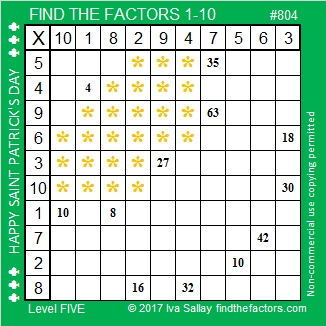# 683 is the 4th Wagstaff Prime

683 is the sum of the five prime numbers from 127 to 149. Can you name those five prime numbers?

Like the number before it, 683 has a relationship with the number 11:

(2¹¹ + 1)/3 = 683. This relationship makes 683 the 4th Wagstaff Prime number. (Notice that 11 is the 4th odd prime number.)

2 raised to an odd prime number has produced many Wagstaff Prime numbers, but not always. For example (2²⁹ + 1)/3 is not a prime number.Print the puzzles or type the solution on this excel file: 12 Factors 2015-11-16

—————————————————————————————————

• 683 is a prime number.
• Prime factorization: 683 is prime.
• The exponent of prime number 683 is 1. Adding 1 to that exponent we get (1 + 1) = 2. Therefore 683 has exactly 2 factors.
• Factors of 683: 1, 683
• Factor pairs: 683 = 1 x 683
• 683 has no square factors that allow its square root to be simplified. √683 ≈ 26.13427.How do we know that 683 is a prime number? If 683 were not a prime number, then it would be divisible by at least one prime number less than or equal to √683 ≈ 26.1. Since 683 cannot be divided evenly by 2, 3, 5, 7, 11, 13, 17, 19, or 23, we know that 683 is a prime number.

—————————————————————————————————

A Logical Approach to solve a FIND THE FACTORS puzzle: Find the column or row with two clues and find their common factor. (None of the factors are greater than 12.)  Write the corresponding factors in the factor column (1st column) and factor row (top row).  Because this is a level three puzzle, you have now written a factor at the top of the factor column. Continue to work from the top of the factor column to the bottom, finding factors and filling in the factor column and the factor row one cell at a time as you go.# 682 Deserves a Lot of Exclamation Points!!!

682 is the sum of the four prime numbers from 163 to 179. 682 is also the sum of the ten prime numbers from 47 to 89.

6 – 8 + 2 = 0 so 682 is divisible by 11.

OEIS.org shared another amazing relationship between the number 682 and the number 11:Besides the obvious inclusion of the digits 6-8-2, notice in the factorial expression that 11 is in each numerator and that 11 is also the sum of the numbers in each denominator.Print the puzzles or type the solution on this excel file: 12 Factors 2015-11-16

—————————————————————————————————

• 682 is a composite number.
• Prime factorization: 682 = 2 x 11 x 31
• The exponents in the prime factorization are 1, 1, and 1. Adding one to each and multiplying we get (1 + 1)(1 + 1)(1 + 1) = 2 x 2 x 2 = 8. Therefore 682 has exactly 8 factors.
• Factors of 682: 1, 2, 11, 22, 31, 62, 341, 682
• Factor pairs: 682 = 1 x 682, 2 x 341, 11 x 62, or 22 x 31
• 682 has no square factors that allow its square root to be simplified. √682 ≈ 26.1151297.—————————————————————————————————# 668 and Level 3

668 is the sum of consecutive prime numbers 331 and 337.

68 is divisible by 4 so 668 and every other number ending in 68 is divisible by 4.

80 + 81 + 82 + 83 + 84 + 85 + 86 + 87 = 668Print the puzzles or type the solution on this excel file: 12 Factors 2015-11-02

—————————————————————————————————

• The first ten multiples of 668 are 668, 1336, 2004, 2672, 3340, 4008, 4676, 5344, 6012, and 6680.
• 668 is a composite number.
• Prime factorization: 668 = 2 x 2 x 167, which can be written 668 = (2^2) x 167
• The exponents in the prime factorization are 2 and 1. Adding one to each and multiplying we get (2 + 1)(1 + 1) = 3 x 2  = 6. Therefore 668 has exactly 6 factors.
• Factors of 668: 1, 2, 4, 167, 334, 668
• Factor pairs: 668 = 1 x 668, 2 x 334, or 4 x 167
• Taking the factor pair with the largest square number factor, we get √668 = (√4)(√167) = 2√167 ≈ 25.845696.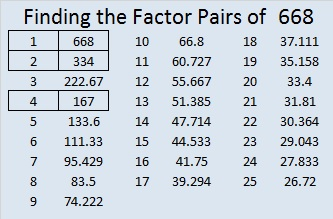—————————————————————————————————

A Logical Approach to solve a FIND THE FACTORS puzzle: Find the column or row with two clues and find their common factor. (None of the factors are greater than 12.)  Write the corresponding factors in the factor column (1st column) and factor row (top row).  Because this is a level three puzzle, you have now written a factor at the top of the factor column. Continue to work from the top of the factor column to the bottom, finding factors and filling in the factor column and the factor row one cell at a time as you go.# 662 More Candy Corn

662 is the sum of the twelve prime numbers from 31 to 79.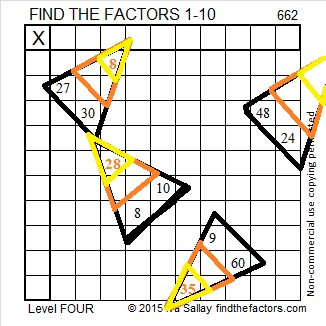Print the puzzles or type the solution on this excel file: 10 Factors 2015-10-26

Here’s the same candy corn puzzle but less colorful.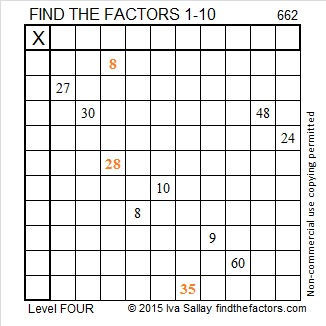—————————————————————————————————

• 662 is a composite number.
• Prime factorization: 662 = 2 x 331
• The exponents in the prime factorization are 1 and 1. Adding one to each and multiplying we get (1 + 1)(1 + 1) = 2 x 2 = 4. Therefore 662 has exactly 4 factors.
• Factors of 662: 1, 2, 331, 662
• Factor pairs: 662 = 1 x 662 or 2 x 331
• 662 has no square factors that allow its square root to be simplified. √662 ≈ 25.72936.—————————————————————————————————Ricardo tweeted the solution to the puzzle so I’m including it here as well.

# 652 and Level 3

652 has 6 factors. 6 is a perfect number because all of its smaller factors, 1, 2, and 3, add up to its largest factor, 6.

The factors of 652 are 1, 2, 4, 163, and 326. The sum of those factors is 496, another perfect number. Note that all of 496’s smaller factors, 1, 2, 4, 8, 16, 31, 62, 124, and 248, add up to 496, its largest factor.

OEIS.org states that 652 is the only known non-perfect number that produces a perfect number in both of those situations.Print the puzzles or type the solution on this excel file: 12 Factors 2015-10-19

—————————————————————————————————

• 652 is a composite number.
• Prime factorization: 652 = 2 x 2 x 163, which can be written 652 = (2^2) x 163
• The exponents in the prime factorization are 2 and 1. Adding one to each and multiplying we get (2 + 1)(1 + 1) = 3 x 2  = 6. Therefore 652 has exactly 6 factors.
• Factors of 652: 1, 2, 4, 163, 326, 652
• Factor pairs: 652 = 1 x 652, 2 x 326, or 4 x 163
• Taking the factor pair with the largest square number factor, we get √652 = (√4)(√163) = 2√163 ≈ 25.53429.—————————————————————————————————

A Logical Approach to solve a FIND THE FACTORS puzzle: Find the column or row with two clues and find their common factor. (None of the factors are greater than 12.)  Write the corresponding factors in the factor column (1st column) and factor row (top row).  Because this is a level three puzzle, you have now written a factor at the top of the factor column. Continue to work from the top of the factor column to the bottom, finding factors and filling in the factor column and the factor row one cell at a time as you go.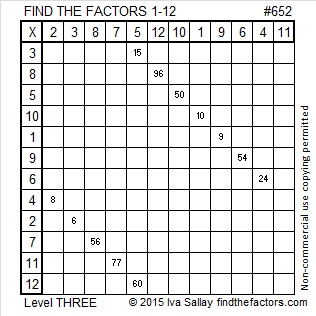# 649 and Level 6

6 – 4 + 9 = 11 so 649 is divisible by 11.

649 is the short leg in exactly three Pythagorean triples. Can you determine which one is a primitive triple, and what are the greatest common factors of each of the two non-primitive triples?

• 649-3540-3599
• 649-19140-19151
• 649-210600-210601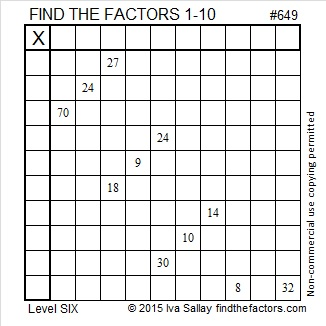Print the puzzles or type the solution on this excel file: 10 Factors 2015-10-12

—————————————————————————————————

• 649 is a composite number.
• Prime factorization: 649 = 11 x 59
• The exponents in the prime factorization are 1 and 1. Adding one to each and multiplying we get (1 + 1)(1 + 1) = 2 x 2 = 4. Therefore 649 has exactly 4 factors.
• Factors of 649: 1, 11, 59, 649
• Factor pairs: 649 = 1 x 649 or 11 x 59
• 649 has no square factors that allow its square root to be simplified. √649 ≈ 25.475478.—————————————————————————————————# 648 and Level 5

648 is the sum of consecutive prime numbers 317 and 331.

The sixth root of 648 begins with 2.941682753. Notice all the digits from 1 to 9 appear somewhere in those nine decimal places. OEIS.org states that 648 is the smallest number that can make that claim.

From Archimedes-lab.org I learned some powerful facts about the number 648:

• 16² – 17² + 18² – 19² + 20² – 21² +22² – 23² + 24² – 25² + 26² – 27² + 28² – 29² + 30² – 31² + 32² = 648
• 48² – 47² + 46² – 45² + 44² – 43² +42² – 41² + 40² – 39² + 38² – 37² + 36² – 35² + 34² – 33² = 648
• (1^2)(2^3)(3^4) = 648
• 18² + 18²  = 648
• (6^3) + (6^3) + (6^3) =648Print the puzzles or type the solution on this excel file: 10 Factors 2015-10-12

—————————————————————————————————

• 648 is a composite number.
• Prime factorization: 648 = 2 x 2 x 2 x 3 x 3 x 3 x 3, which can be written 648 = (2^3) x (3^4)
• The exponents in the prime factorization are 3 and 4. Adding one to each and multiplying we get (3 + 1)(4 + 1) = 4 x 5 = 20. Therefore 648 has exactly 20 factors.
• Factors of 648: 1, 2, 3, 4, 6, 8, 9, 12, 18, 24, 27, 36, 54, 72, 81, 108, 162, 216, 324, 648
• Factor pairs: 648 = 1 x 648, 2 x 324, 3 x 216, 4 x 162, 6 x 108, 8 x 81, 9 x 72, 12 x 54, 18 x 36, or 24 x 27
• Taking the factor pair with the largest square number factor, we get √648 = (√324)(√2) = 18√2 ≈ 25.455844122…—————————————————————————————————# 639 and Level 4

639 is the sum of the 20 prime numbers from 2 to 71, its largest prime factor.

639 is made from 3 numbers that are divisible by 3 so 639 is divisible by 3 AND by 9.

Puzzle #639 has a tricky clue in it, but I’m sure you can still solve it.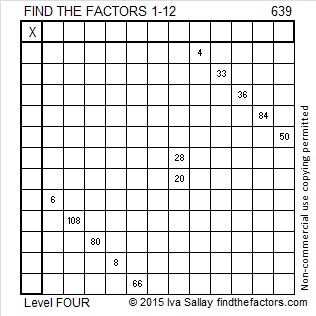Print the puzzles or type the solution on this excel file: 12 Factors 2015-10-05

—————————————————————————————————

• 639 is a composite number.
• Prime factorization: 639 = 3 x 3 x 71, which can be written 639 = (3^2) x 71
• The exponents in the prime factorization are 2 and 1. Adding one to each and multiplying we get (2 + 1)(1 + 1) = 3 x 2  = 6. Therefore 639 has exactly 6 factors.
• Factors of 639: 1, 3, 9, 71, 213, 639
• Factor pairs: 639 = 1 x 639, 3 x 213, or 9 x 71
• Taking the factor pair with the largest square number factor, we get √639 = (√9)(√71) = 3√71 ≈ 25.278449.—————————————————————————————————